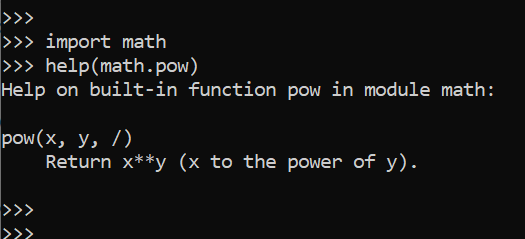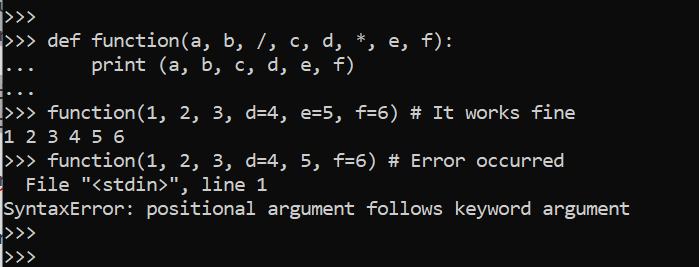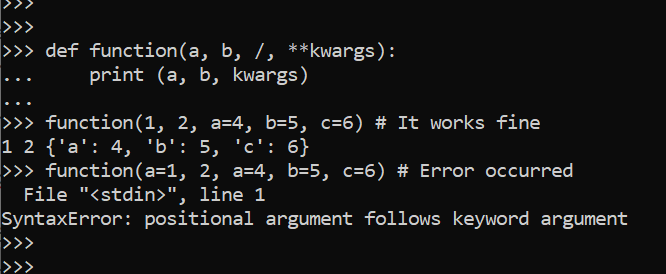Related Articles

# Positional-only Parameter in Python3.8

• Last Updated : 03 Jul, 2020

Python introduces the new function syntax in Python3.8.2 Version, Where we can introduce the `/` forward slash to compare the positional only parameter which comes before the `/` slash and parameters that comes after `*` is keyword only arguments. Rest of the arguments that are come between `/` and `*` can be either positional or keyword type of argument.
That means we can combine positional arguments and regular arguments in such a way all the non-positional argument comes after `/` slash.

Syntax:

Attention geek! Strengthen your foundations with the Python Programming Foundation Course and learn the basics.

To begin with, your interview preparations Enhance your Data Structures concepts with the Python DS Course. And to begin with your Machine Learning Journey, join the Machine Learning - Basic Level Course

```def function(a, b, /, c, d, *, e, f):
# Function Body
pass
```

Where a and b are positional argument, c and d can be either positional or keyword or e and f are strictly keyword type argument.

In the image given below, we can see that power function is a builtin function in Python’s math library and this function uses `/` slash to enable the positional only argument and now we can implement the same functionality with the help of this version.Example #1 :
In this example we can see that by using positional only argument we can implement the function with the fixed position as we can say only in built-in functions before this python version. With the help of this, we are able to make our program more robust.

 `# Positional-Only argument ``def` `function(a, b, ``/``, c, d, ``*``, e, f):``    ``print` `(a, b, c, d, e, f)`` ` `function(``1``, ``2``, ``3``, d ``=` `4``, e ``=` `5``, f ``=` `6``) ``# It works fine``function(``1``, ``2``, ``3``, d ``=` `4``, ``5``, f ``=` `6``) ``# Error occurred`

Output :Example #2 :

 `# Positional-Only argument ``def` `function(a, b, ``/``, ``*``*``kwargs):``    ``print` `(a, b, kwargs)`` ` `function(``1``, ``2``, a ``=` `4``, b ``=` `5``, c ``=` `6``) ``# It works fine``function(a ``=` `1``, ``2``, a ``=` `4``, b ``=` `5``, c ``=` `6``) ``# Error occurred`

Output :My Personal Notes arrow_drop_up